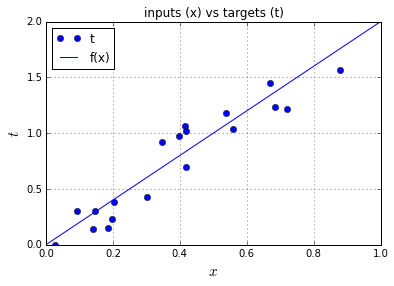# Good Articles to learn how to implement a neural network 1

This series of post will list some good articles about how to implement a neural network. Thanks for the authors for the excellent work.
If you are the author and you don’t want your articles listed here. Please email to learn4master, we will remove it from the site.

# How to implement a neural network Part 1

This page is part of a 5 (+2) parts tutorial on how to implement a simple neural network model. You can find the links to the rest of the tutorial here:

The tutorials are generated from Python 2 IPython Notebook files, which will be linked to at the end of each chapter so that you can adapt and run the examples yourself. The neural networks themselves are implemented using the Python NumPy library which offers efficient implementations of linear algebra functions such as vector and matrix multiplications. Illustrative plots are generated using Matplotlib . If you want to run these examples yourself and don’t have Python with the necessary libraries installed I recommend to download and install Anaconda Python , which is a free Python distribution that contains all the libraries you need to run these tutorials, and is used to create these tutorials.

The code input cells in this blog can be collapsed or expanded by clicking on the button in the top right of each cell.

A version of this tutorial is also available in Chinese thanks to Mingming Chen .

## Linear regression

This first part will cover:

All this will be illustrated with the help of the simplest neural network possible: a 1 input 1 output linear regression model that has the goal to predict the target value t

from the input value x. The network is defined as having an input x which gets transformed by the weight w to generate the output y by the formula y=xw, and where y needs to approximate the targets t

as good as possible as defined by a cost function. This network can be represented graphically as:In regular neural networks, we typically have multiple layers, non-linear activation functions, and a bias for each node. In this tutorial, we only have one layer with one weight parameter w

, no activation function on the output, and no bias. In simple linear regression the parameter w and bias are typically combined into the parameter vector β where bias is the y-intercept and w

is the slope of the regression line. In linear regression, these parameters are typically fitted via the least squares method .

In this tutorial, we will approximate the targets t

with the outputs of the model y by minimizing the squared error cost function (= squared Euclidian distance). The squared error cost function is defined as ty2

. The minimization of the cost will be done with the gradient descent optimization algorithm which is typically used in training of neural networks.

The notebook starts out with importing the libraries we need:

In :

## Define the target function

In this example, the targets t

will be generated from a function f and additive gaussian noise sampled from (0,0.2), where is the normal distribution with mean 0 and variance 0.2. f is defined as f(x)=x2, with x the input samples, slope 2 and intercept 0. t is f(x)+(0,0.2)

.

We will sample 20 input samples x

from the uniform distribution between 0 and 1, and then generate the target output values t by the process described above. These resulting inputs x and targets t are plotted against each other in the figure below together with the original f(x) line without the gaussian noise. Note that x is a vector of individual input samples xi, and that t is a corresponding vector of target values ti

.

In :

In :## Define the cost function

We will optimize the model y=xw

by tuning parameter w so that the squared error cost along all samples is minimized. The squared error cost is defined as ξ=Ni=1tiyi2, with N the number of samples in the training set. The optimization goal is thus: argminwNi=1tiyi2

.
Notice that we take the sum of errors over all samples, which is known as batch training. We could also update the parameters based upon one sample at a time, which is known as online training.

This cost function for variable w

is plotted in the figure below. The value w=2 is at the minimum of the cost function (bottom of the parabola), this value is the same value as the slope we choose for f(x)

. Notice that this function is convex and that there is only one minimum: the global minimum. While every squared error cost function for linear regression is convex, this is not the case for other models and other cost functions.

The neural network model is implemented in the nn(x, w) function, and the cost function is implemented in the cost(y, t) function.

In :